# Frank Solutions for Class 10 Maths Chapter 17 Circles

Frank Solutions for Class 10 Maths Chapter 17 Circles are given here. Students can refer to these Frank Solutions, which help in gaining better knowledge through proper guidance. Solving the exercises in each chapter will ensure that students score good marks in the exams. Our solution module utilises various shortcut tips and practical examples to explain all the exercise questions in simple and easily understandable language. If you wish to secure an excellent score, solving Frank Solutions for Class 10 Maths Chapter 17 is a must.

Chapter 17 – Circles explains a circle, which is the set of all points in the plane that are a fixed distance from a fixed point. Here, we have provided the solutions for Class 10 Maths Chapter 17, which are solved in detail. Subject experts at BYJU’S have designed these solutions in a very lucid and clear manner that helps students solve problems in the most efficient possible ways. Download the PDF of Class 10 Chapter 17 from the link below.

## Download the PDF of Frank Solutions for Class 10 Maths Chapter 17 Circles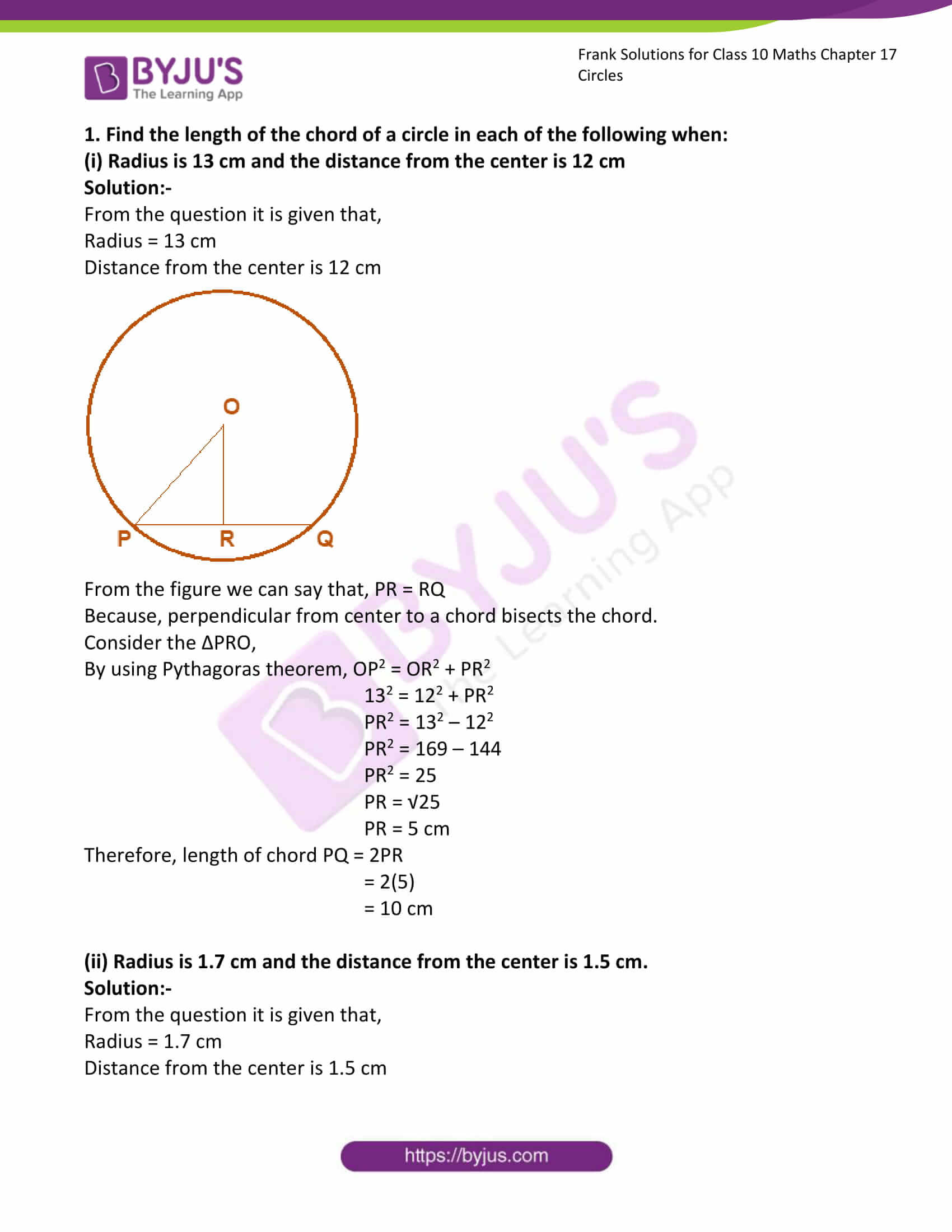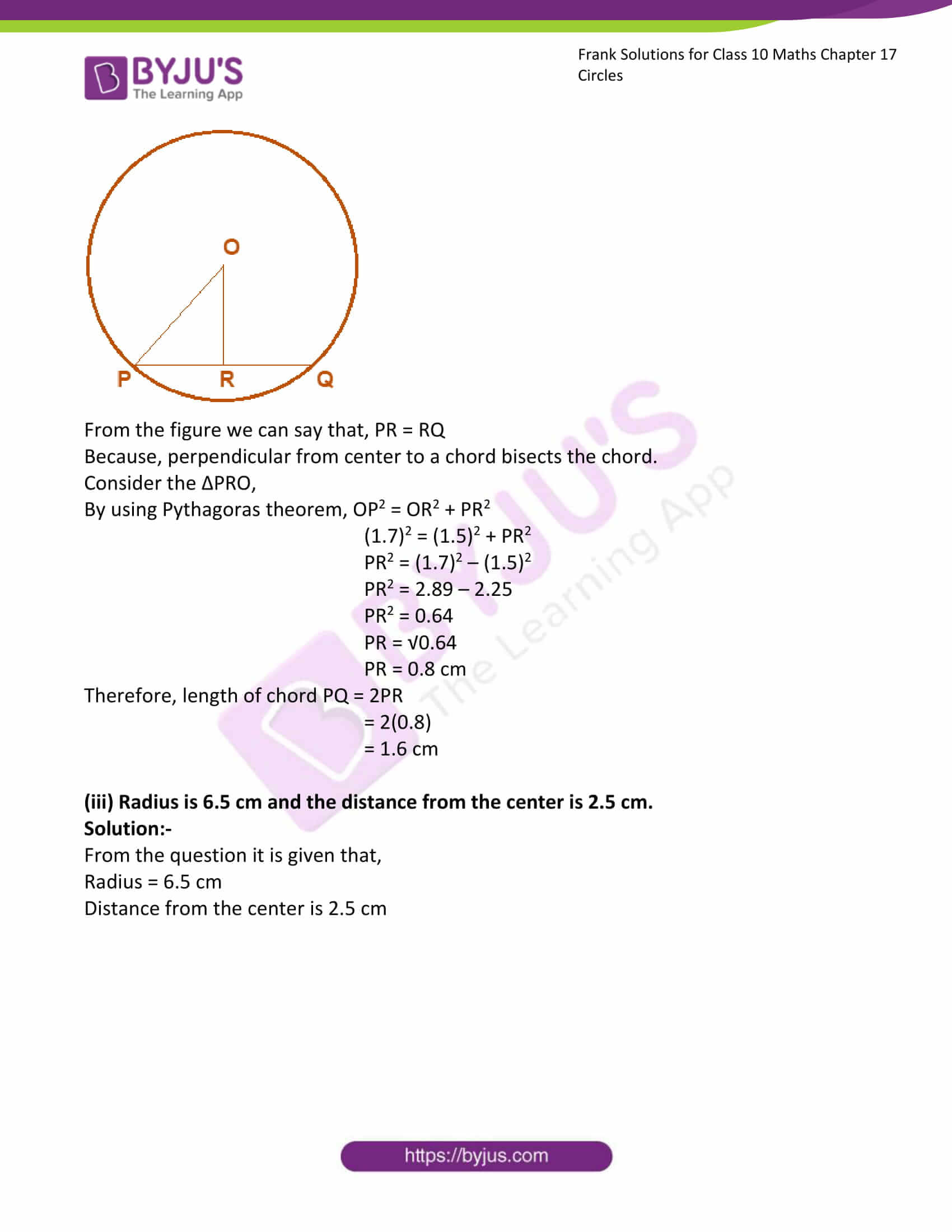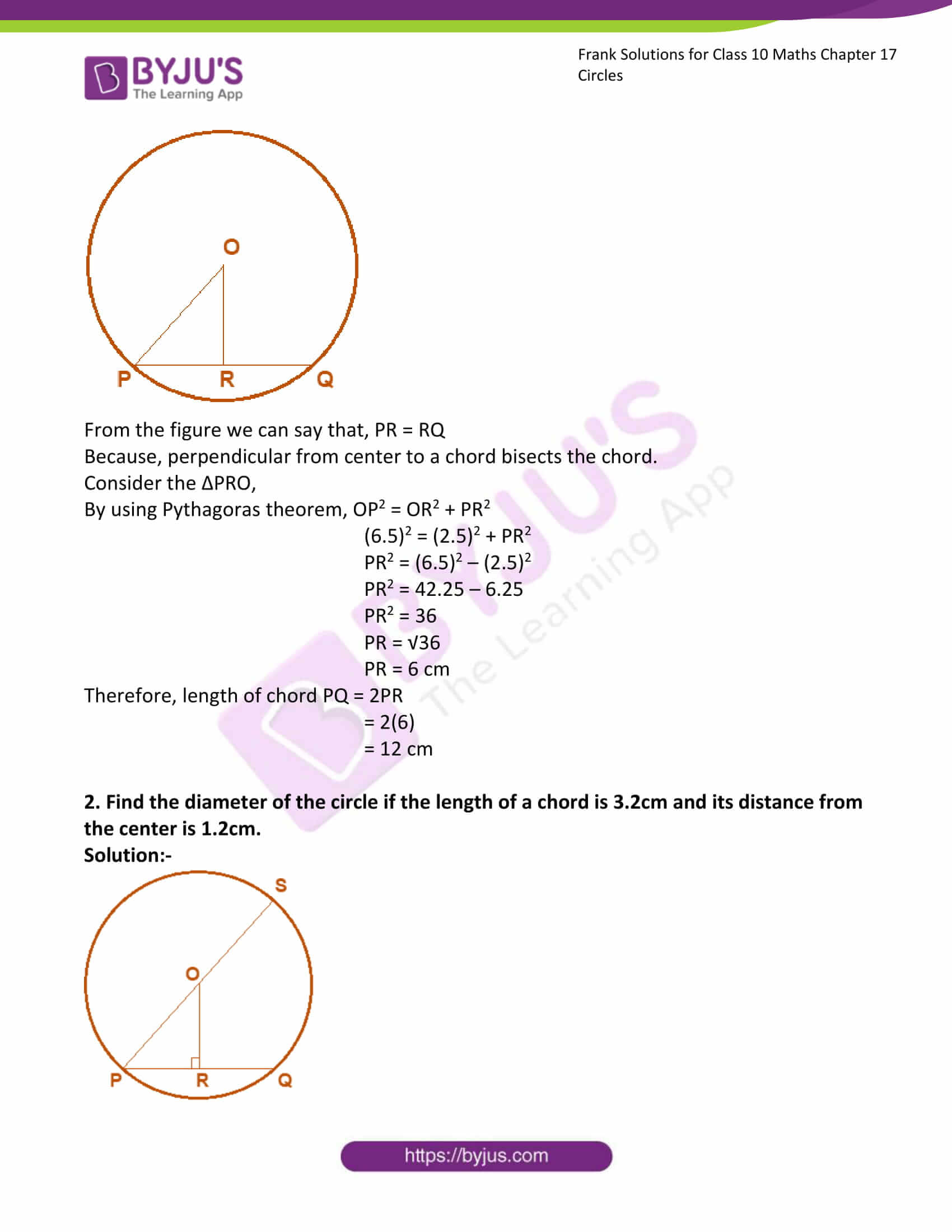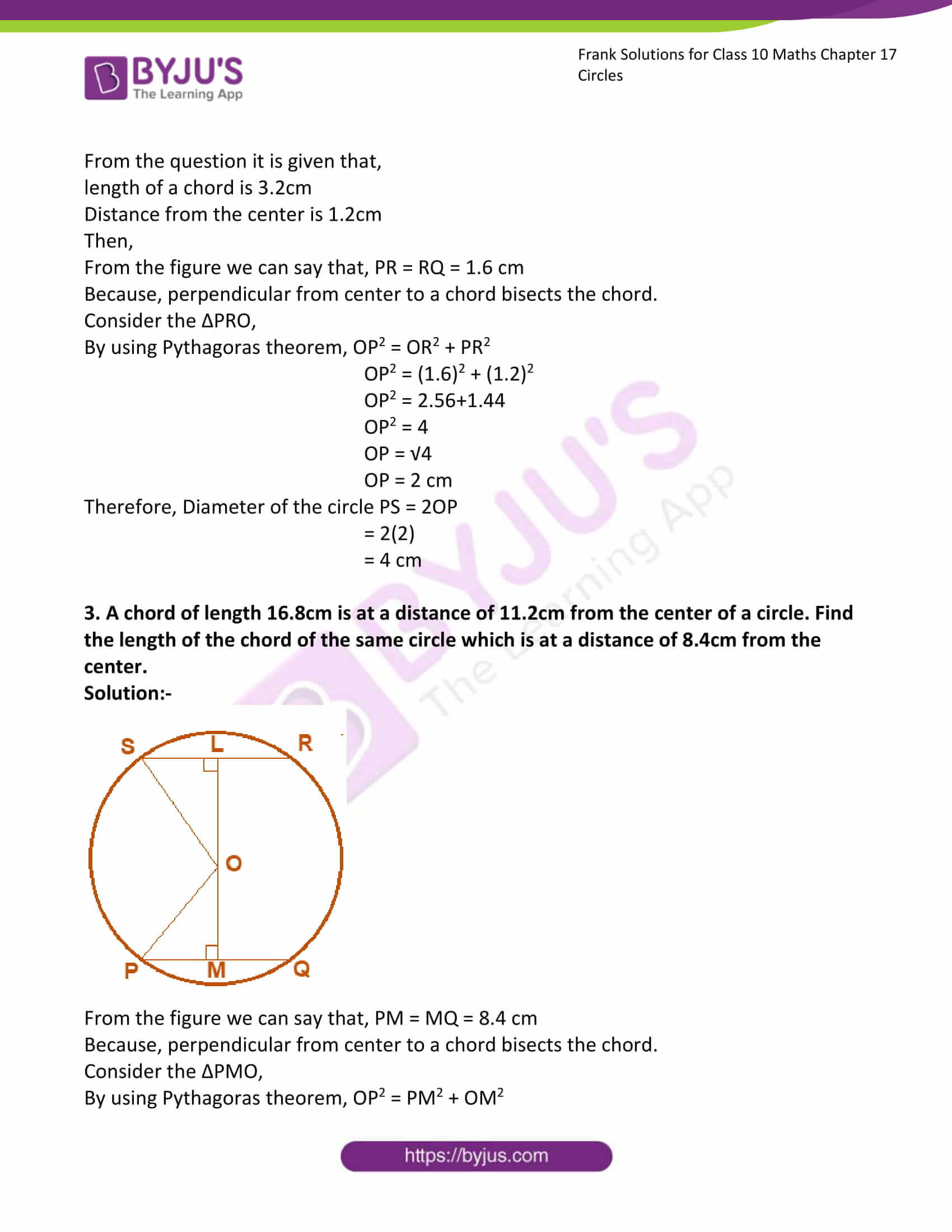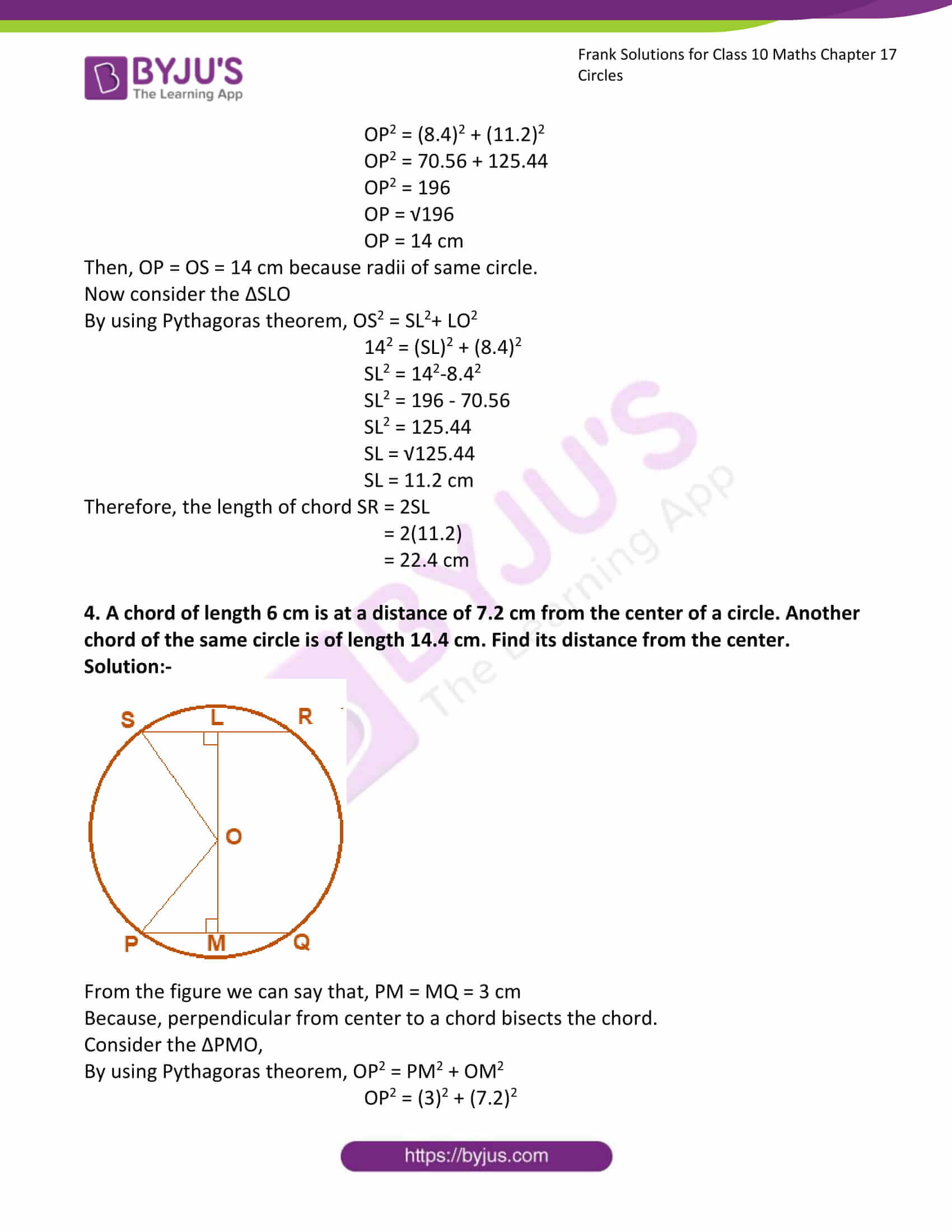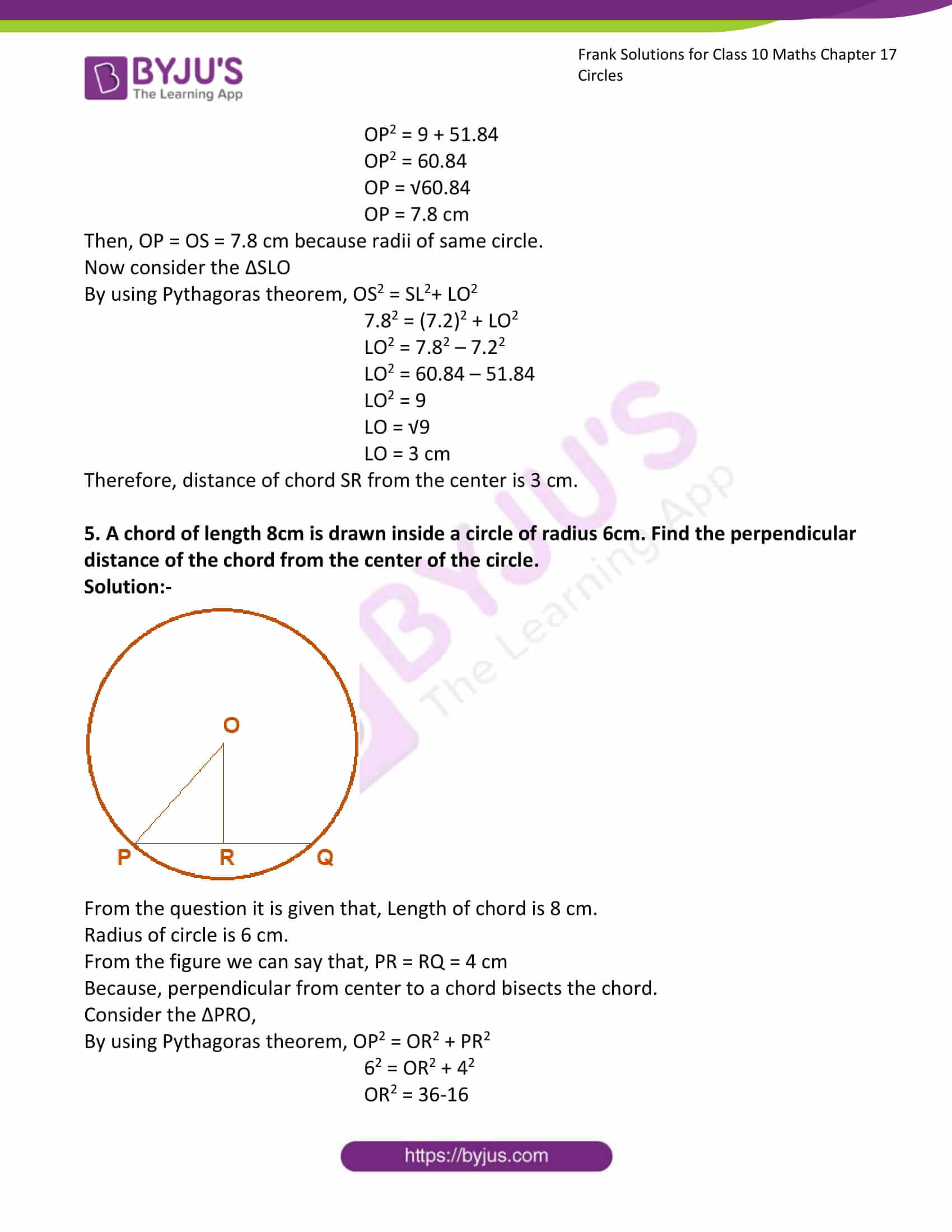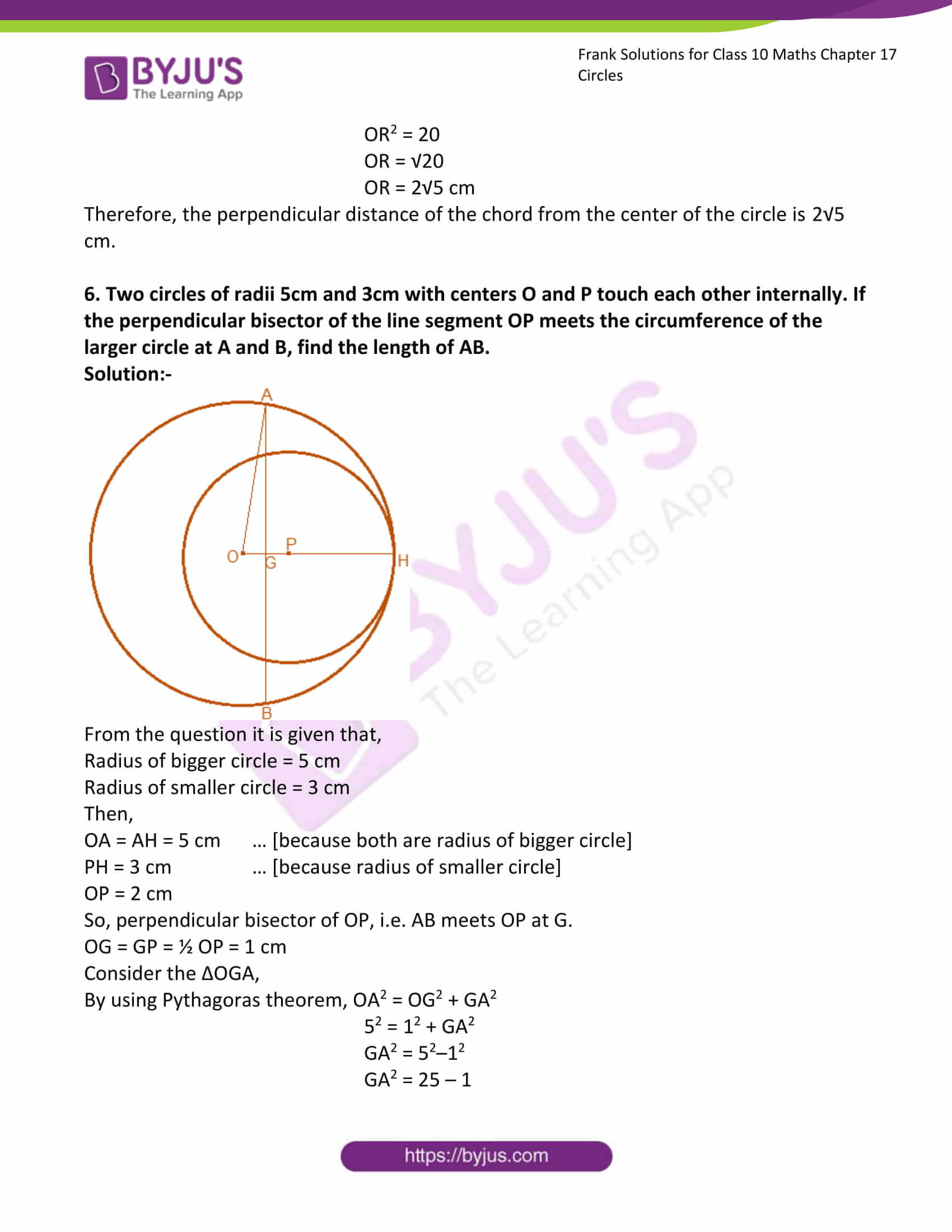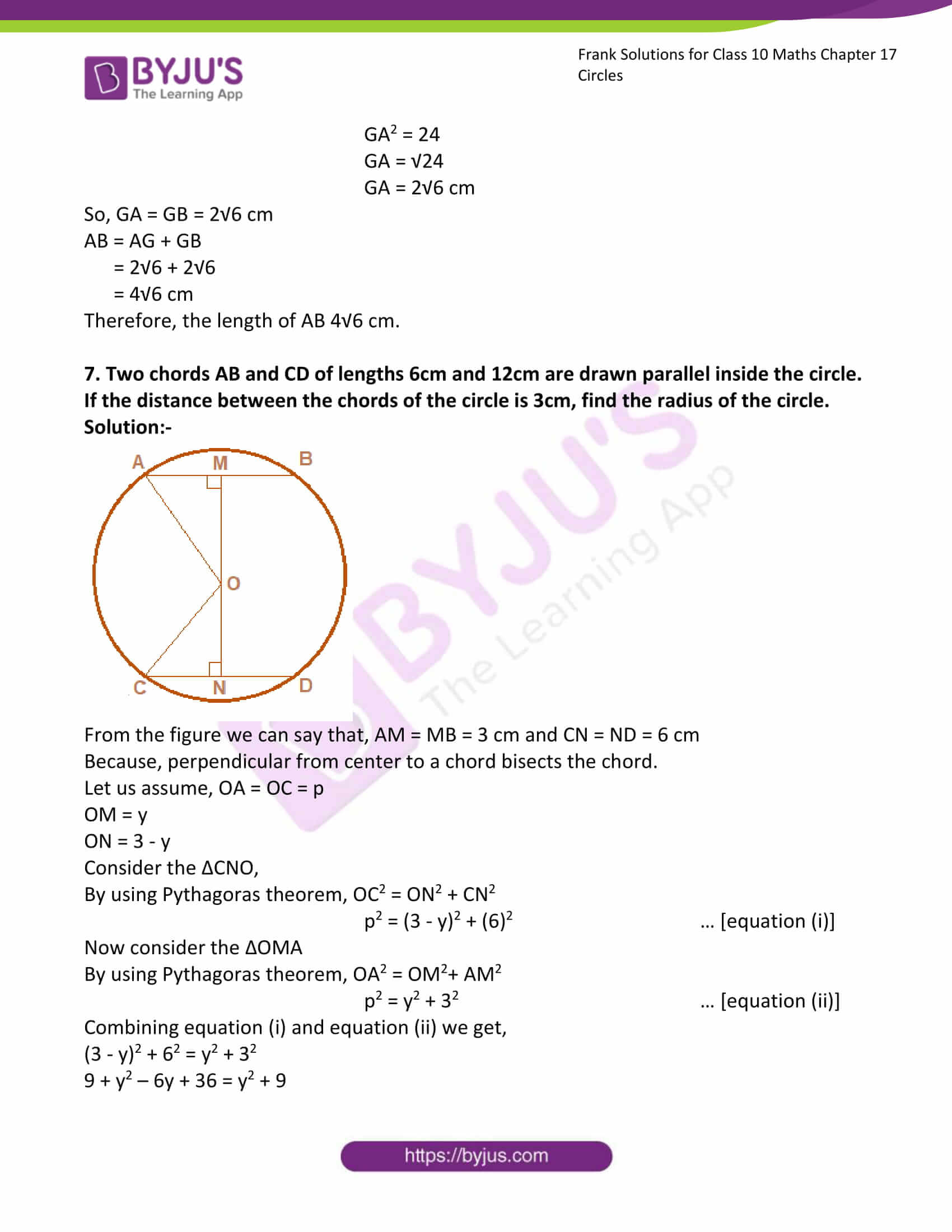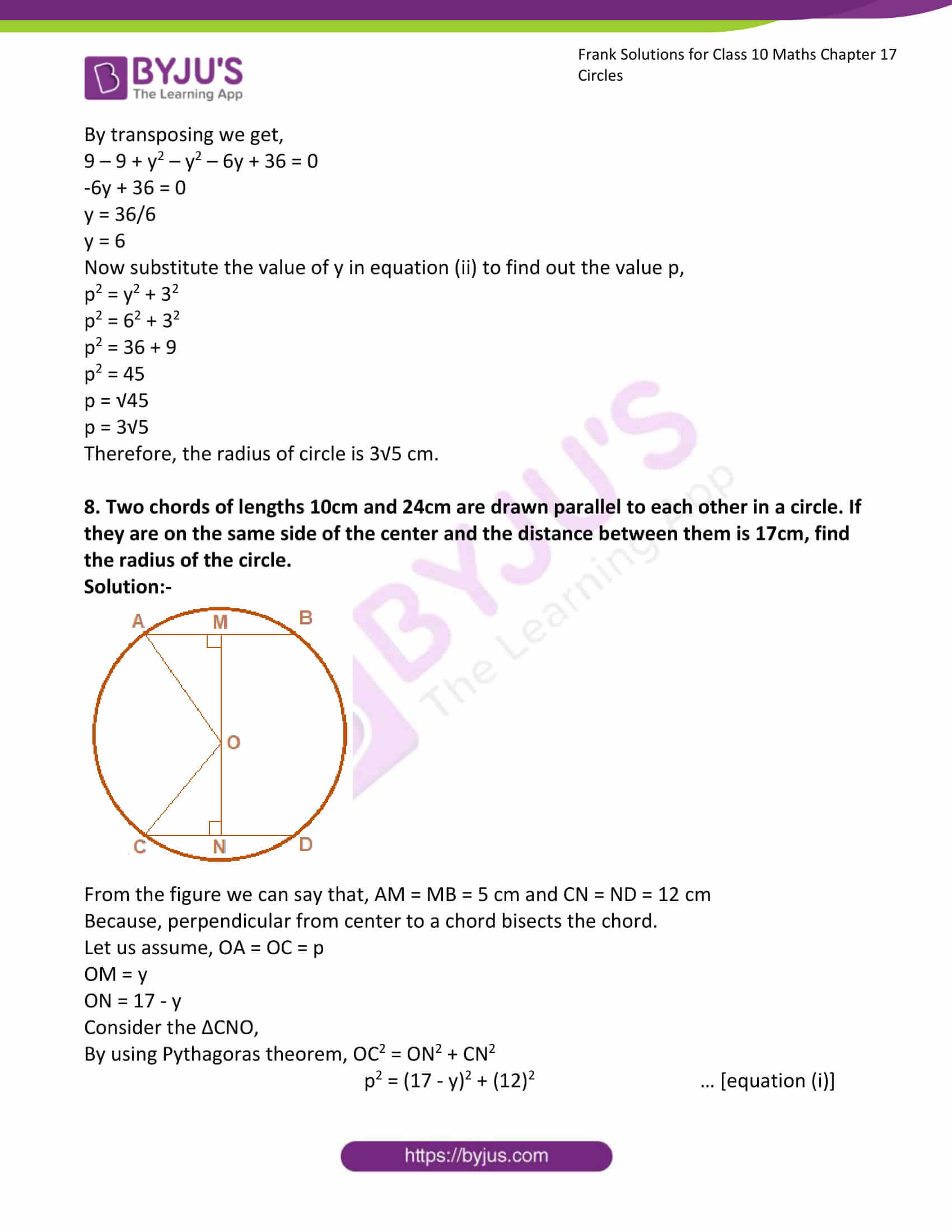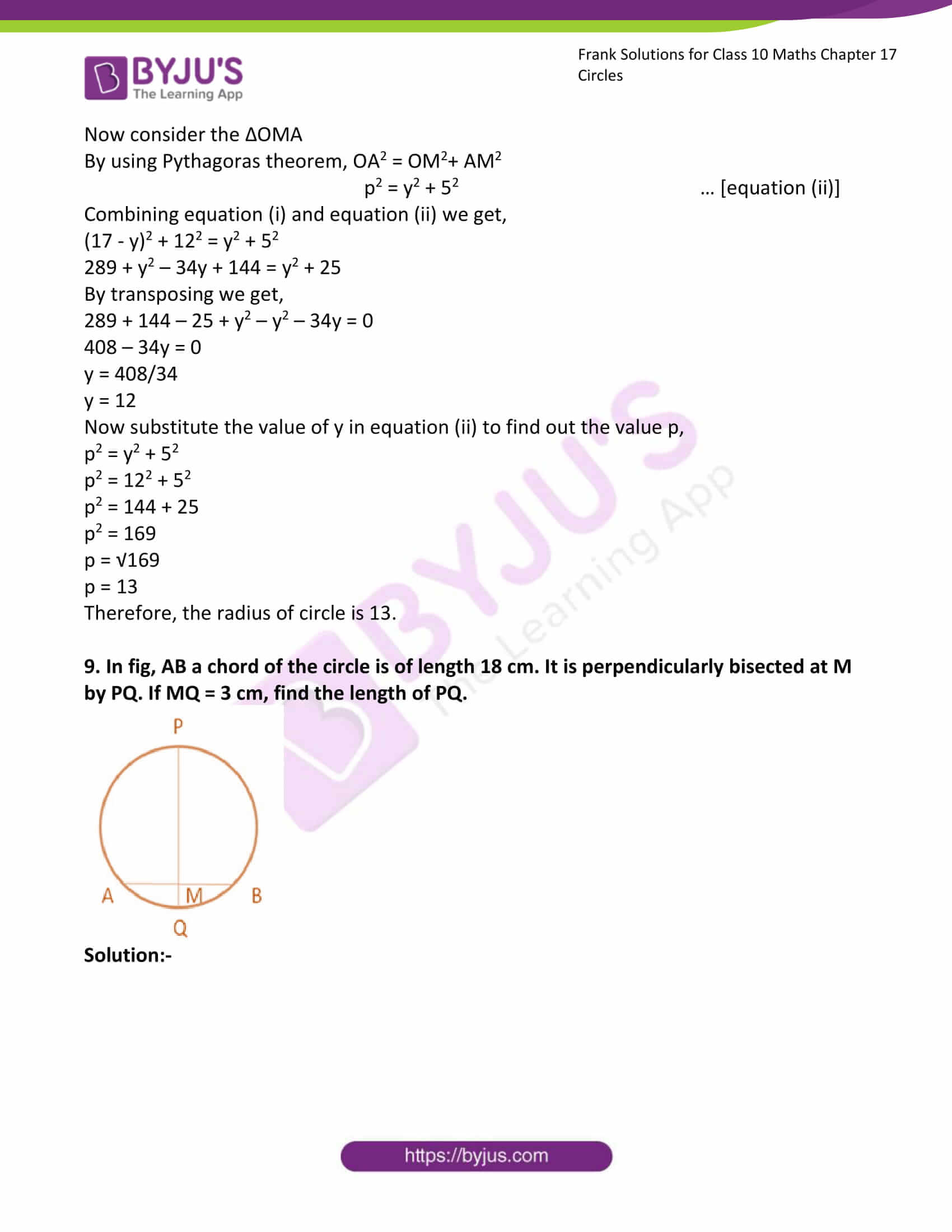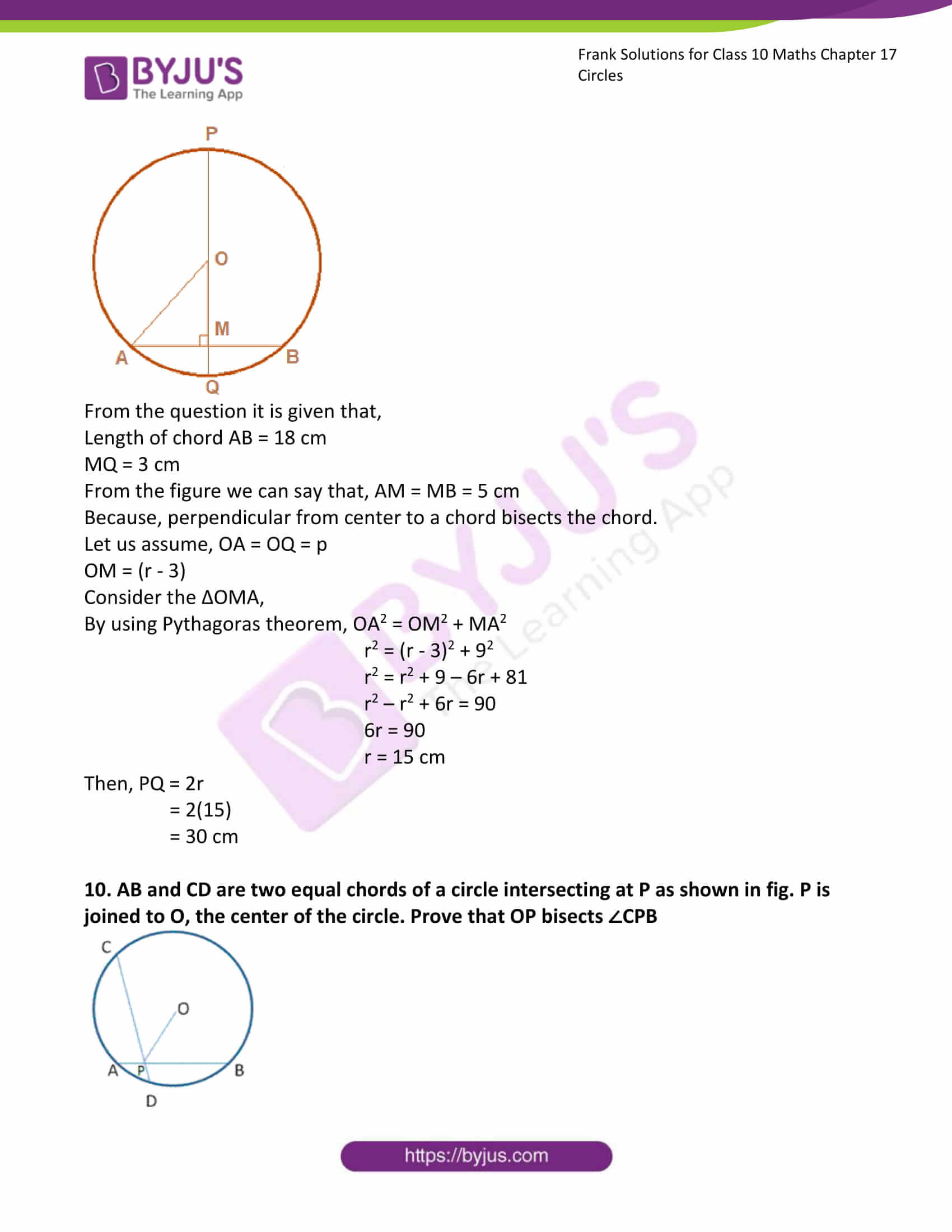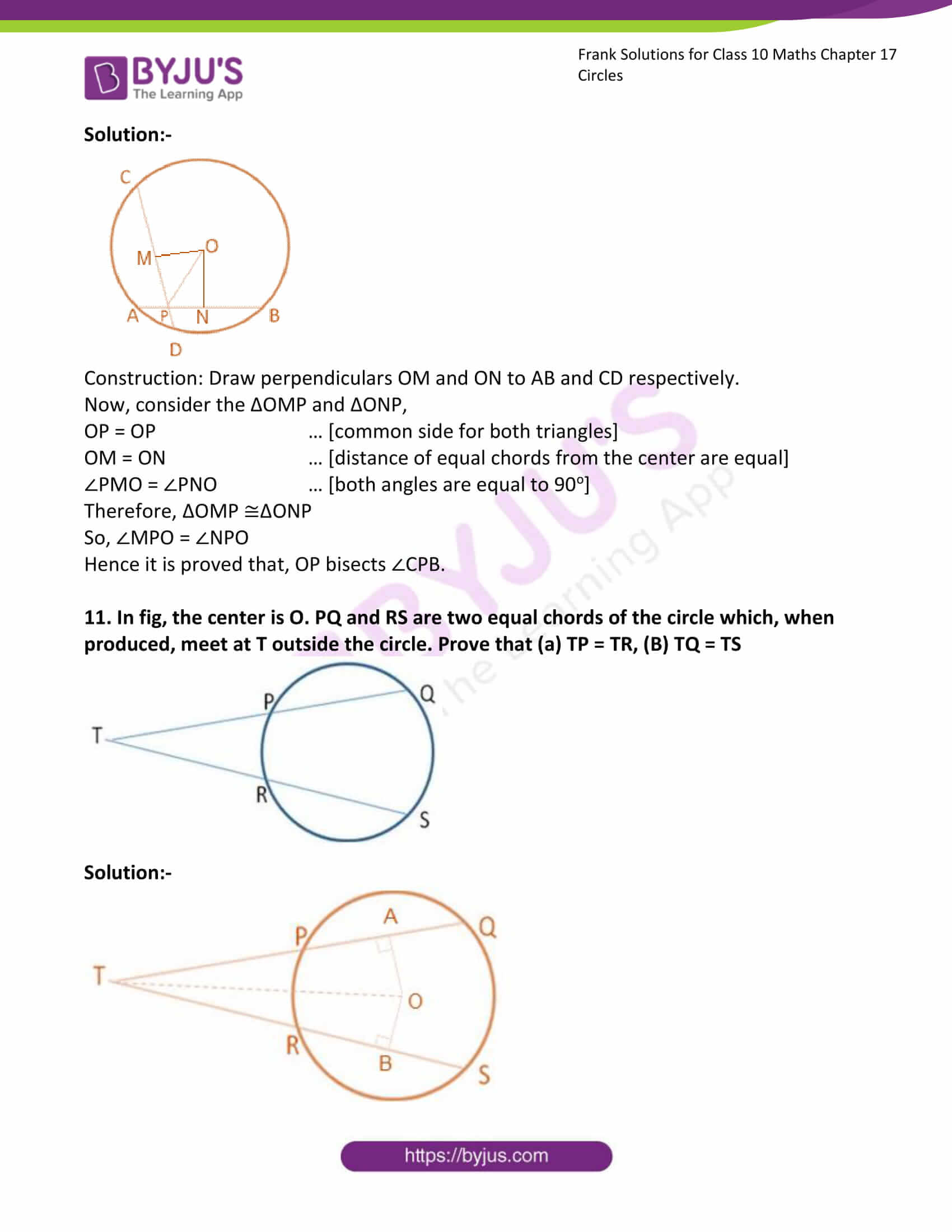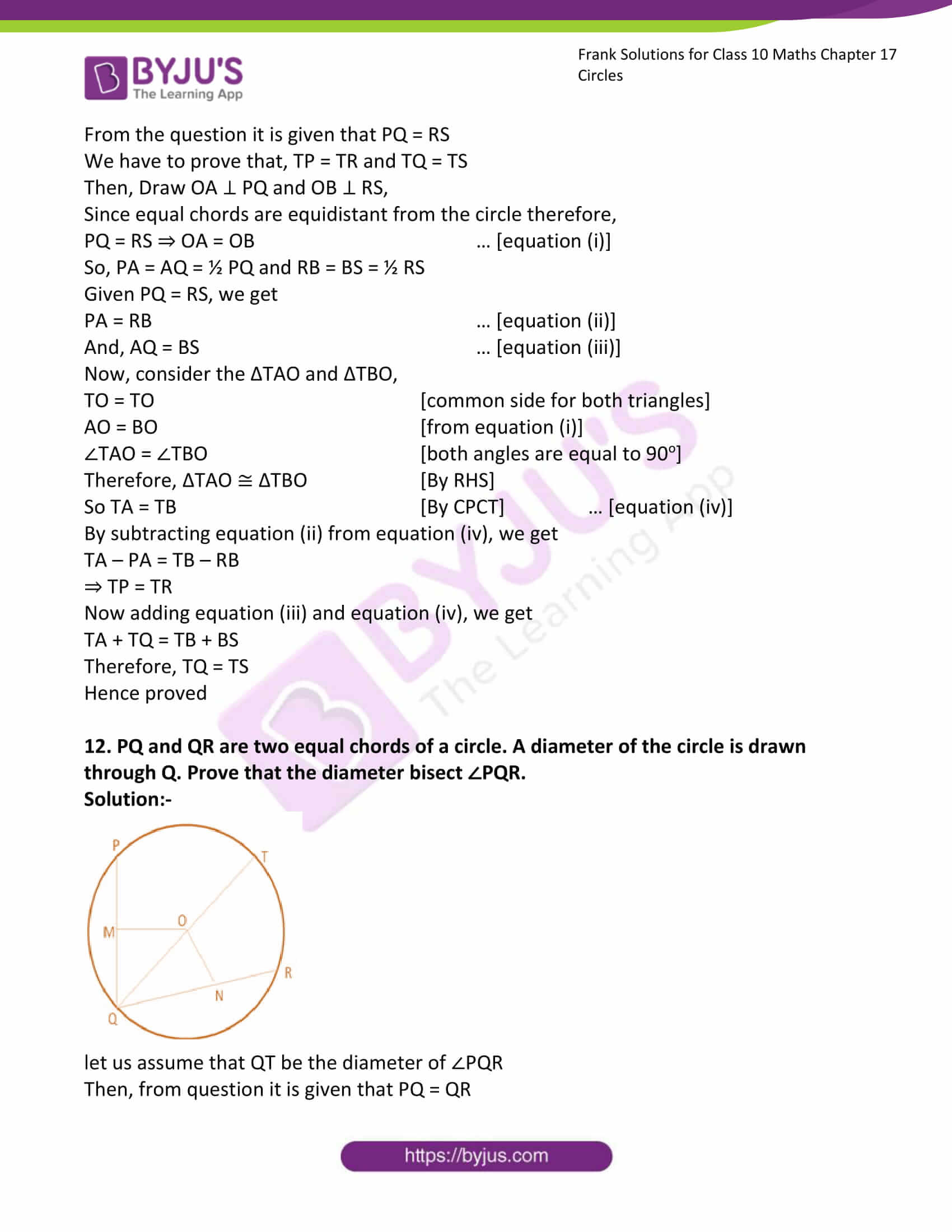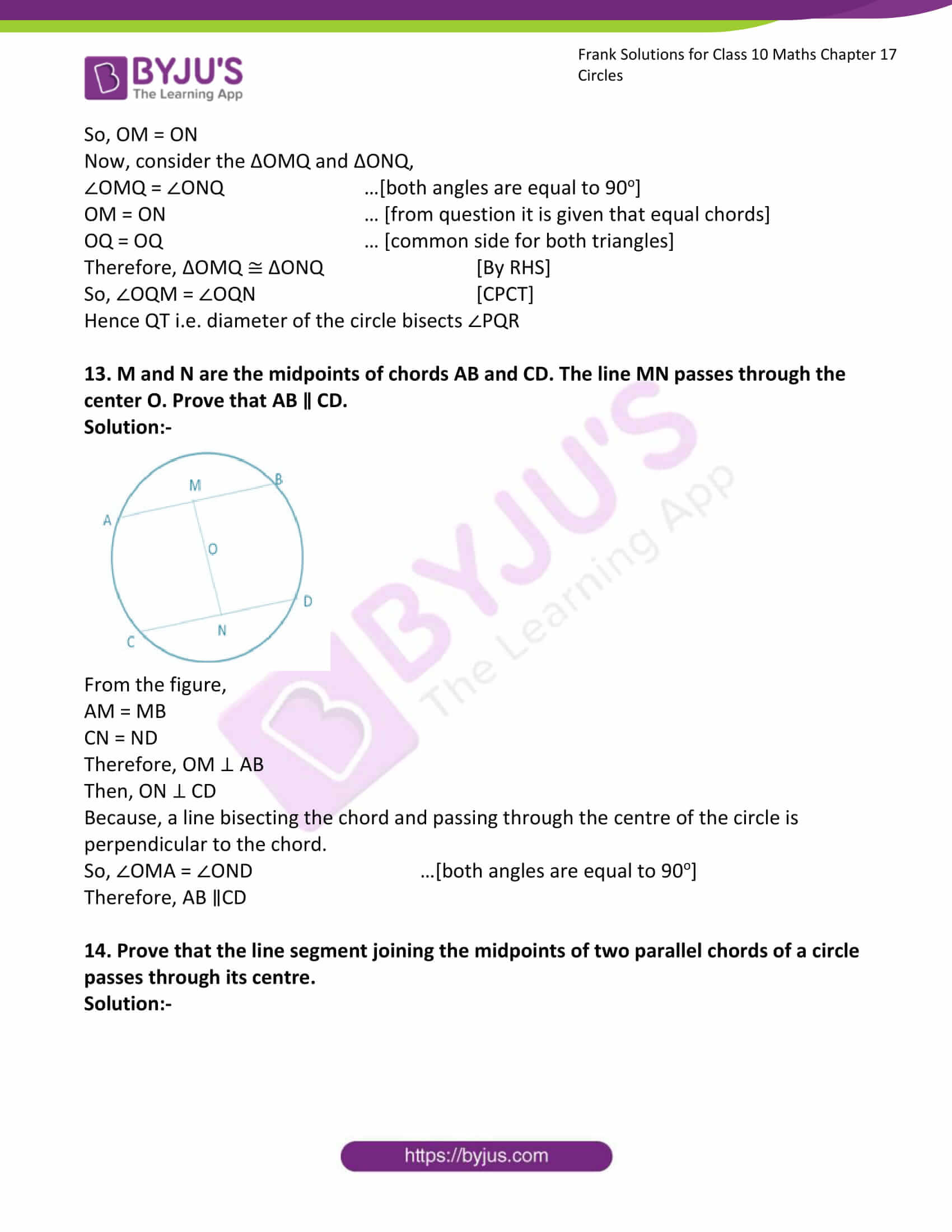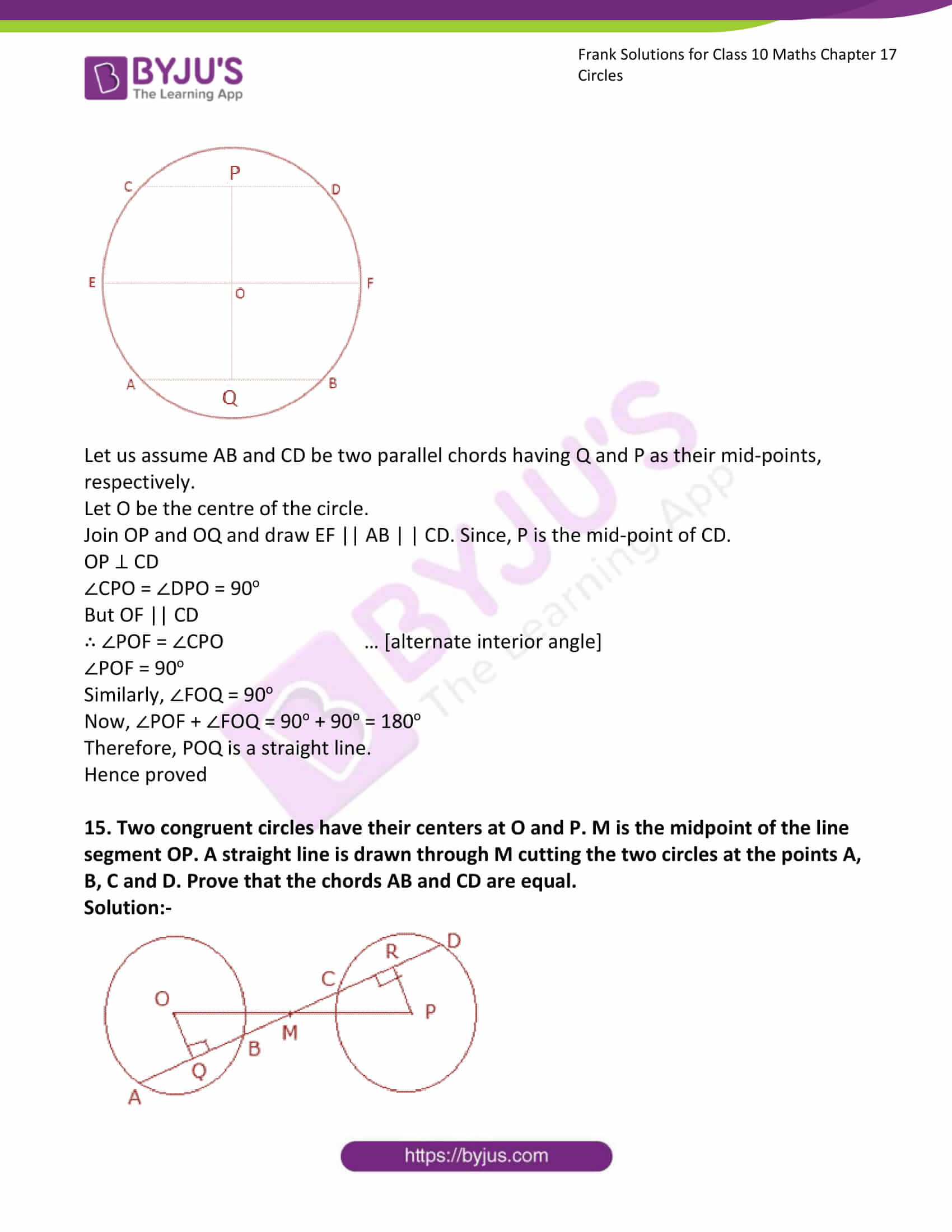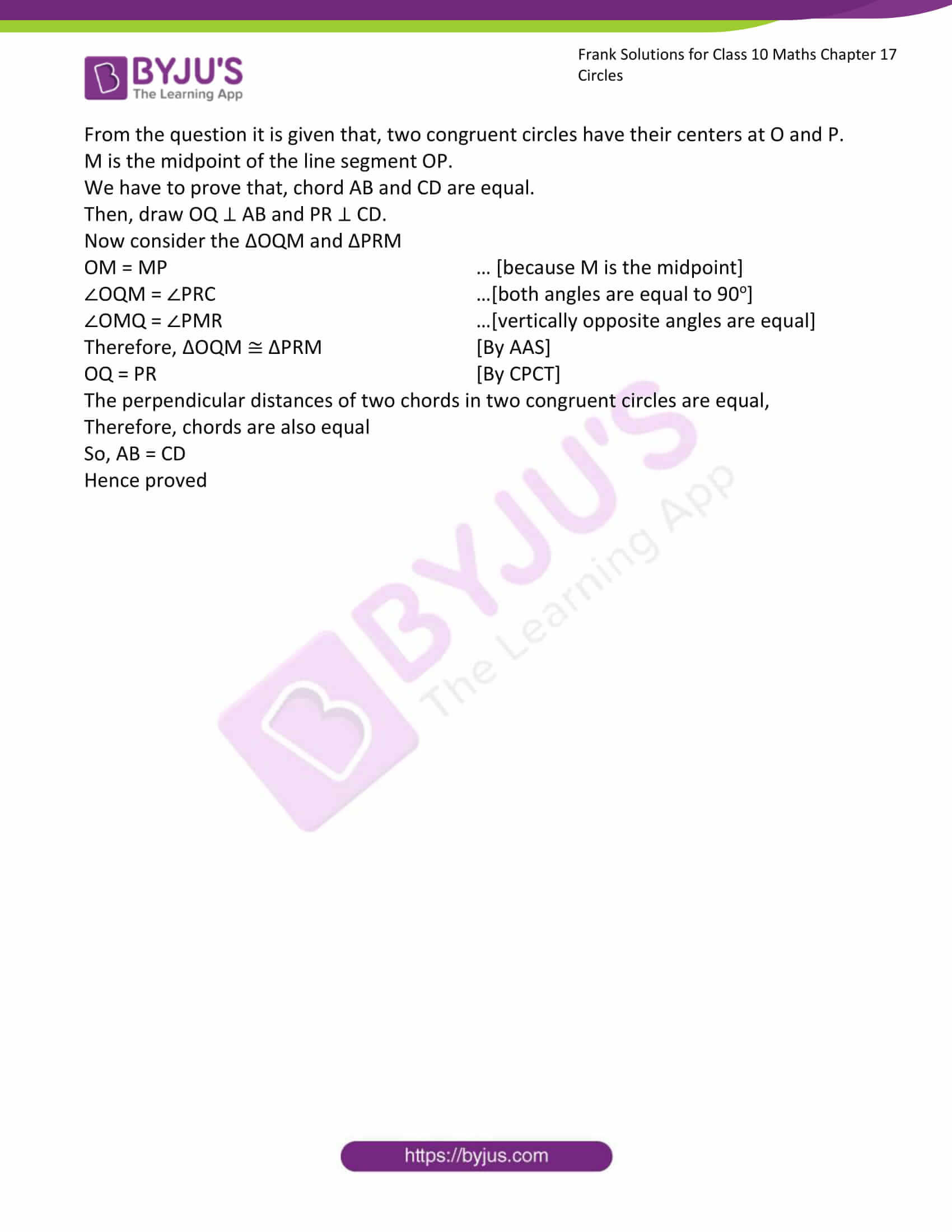### Access Answers to Frank Solutions for Class 10 Maths Chapter 17 Circles

1. Find the length of the chord of a circle in each of the following when:

(i) Radius is 13 cm, and the distance from the centre is 12 cm

Solution:-

From the question, it is given that,

Distance from the centre is 12 cm.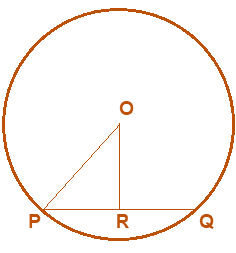From the figure, we can say that PR = RQ

Because perpendicular from the centre to a chord bisects the chord.

Consider the ΔPRO,

By using Pythagoras theorem, OP2 = OR2 + PR2

132 = 122 + PR2

PR2 = 132 – 122

PR2 = 169 – 144

PR2 = 25

PR = √25

PR = 5 cm

Therefore, length of chord PQ = 2PR

= 2(5)

= 10 cm

(ii) Radius is 1.7 cm, and the distance from the centre is 1.5 cm.

Solution:-

From the question, it is given that,

Distance from the centre is 1.5 cmFrom the figure, we can say that PR = RQ

Because perpendicular from centre to a chord bisects the chord.

Consider the ΔPRO,

By using Pythagoras theorem, OP2 = OR2 + PR2

(1.7)2 = (1.5)2 + PR2

PR2 = (1.7)2 – (1.5)2

PR2 = 2.89 – 2.25

PR2 = 0.64

PR = √0.64

PR = 0.8 cm

Therefore, the length of chord PQ = 2PR

= 2(0.8)

= 1.6 cm

(iii) Radius is 6.5 cm, and the distance from the centre is 2.5 cm.

Solution:-

From the question, it is given that,

Distance from the centre is 2.5 cmFrom the figure, we can say that PR = RQ

Because perpendicular from centre to a chord bisects the chord.

Consider the ΔPRO,

By using Pythagoras theorem, OP2 = OR2 + PR2

(6.5)2 = (2.5)2 + PR2

PR2 = (6.5)2 – (2.5)2

PR2 = 42.25 – 6.25

PR2 = 36

PR = √36

PR = 6 cm

Therefore, the length of chord PQ = 2PR

= 2(6)

= 12 cm

2. Find the diameter of the circle if the length of a chord is 3.2cm and its distance from the centre is 1.2cm.

Solution:-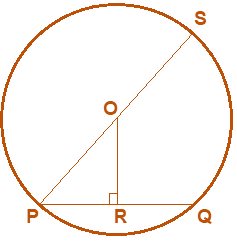From the question, it is given that,

length of a chord is 3.2cm

Distance from the centre is 1.2cm

Then,

From the figure, we can say that PR = RQ = 1.6 cm

Because perpendicular from centre to a chord bisects the chord.

Consider the ΔPRO,

By using Pythagoras theorem, OP2 = OR2 + PR2

OP2 = (1.6)2 + (1.2)2

OP2 = 2.56+1.44

OP2 = 4

OP = √4

OP = 2 cm

Therefore, the diameter of the circle PS = 2OP

= 2(2)

= 4 cm

3. A chord of length 16.8cm is at a distance of 11.2cm from the centre of a circle. Find the length of the chord of the same circle which is at a distance of 8.4cm from the centre.

Solution:-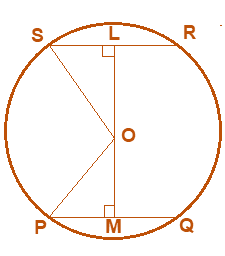From the figure, we can say that PM = MQ = 8.4 cm

Because perpendicular from the centre to a chord bisects the chord.

Consider the ΔPMO,

By using Pythagoras theorem, OP2 = PM2 + OM2

OP2 = (8.4)2 + (11.2)2

OP2 = 70.56 + 125.44

OP2 = 196

OP = √196

OP = 14 cm

Then, OP = OS = 14 cm because radii of same circle.

Now consider the ΔSLO

By using Pythagoras theorem, OS2 = SL2+ LO2

142 = (SL)2 + (8.4)2

SL2 = 142-8.42

SL2 = 196 – 70.56

SL2 = 125.44

SL = √125.44

SL = 11.2 cm

Therefore, the length of chord SR = 2SL

= 2(11.2)

= 22.4 cm

4. A chord of length 6 cm is at a distance of 7.2 cm from the centre of a circle. Another chord of the same circle is of length 14.4 cm. Find its distance from the centre.

Solution:-From the figure, we can say that, PM = MQ = 3 cm

Because, perpendicular from centre to a chord bisects the chord.

Consider the ΔPMO,

By using Pythagoras theorem, OP2 = PM2 + OM2

OP2 = (3)2 + (7.2)2

OP2 = 9 + 51.84

OP2 = 60.84

OP = √60.84

OP = 7.8 cm

Then, OP = OS = 7.8 cm because radii of same circle.

Now consider the ΔSLO

By using Pythagoras theorem, OS2 = SL2+ LO2

7.82 = (7.2)2 + LO2

LO2 = 7.82 – 7.22

LO2 = 60.84 – 51.84

LO2 = 9

LO = √9

LO = 3 cm

Therefore, distance of chord SR from the centre is 3 cm.

5. A chord of length 8cm is drawn inside a circle of radius 6cm. Find the perpendicular distance of the chord from the centre of the circle.

Solution:-From the question, it is given that, Length of chord is 8 cm.

Radius of circle is 6 cm.

From the figure we can say that, PR = RQ = 4 cm

Because, perpendicular from centre to a chord bisects the chord.

Consider the ΔPRO,

By using Pythagoras theorem, OP2 = OR2 + PR2

62 = OR2 + 42

OR2 = 36-16

OR2 = 20

OR = √20

OR = 2√5 cm

Therefore, the perpendicular distance of the chord from the centre of the circle is 2√5 cm.

6. Two circles of radii 5cm and 3cm with centres O and P touch each other internally. If the perpendicular bisector of the line segment OP meets the circumference of the larger circle at A and B, find the length of AB.

Solution:-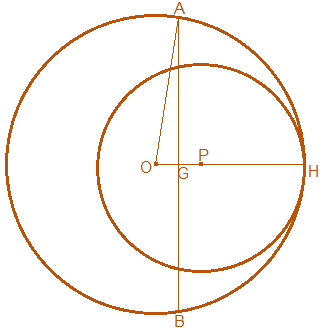From the question, it is given that,

Radius of bigger circle = 5 cm

Radius of smaller circle = 3 cm

Then,

OA = AH = 5 cm … [because both are radius of bigger circle]

PH = 3 cm … [because radius of smaller circle]

OP = 2 cm

So, perpendicular bisector of OP, i.e. AB meets OP at G.

OG = GP = ½ OP = 1 cm

Consider the ΔOGA,

By using Pythagoras theorem, OA2 = OG2 + GA2

52 = 12 + GA2

GA2 = 52–12

GA2 = 25 – 1

GA2 = 24

GA = √24

GA = 2√6 cm

So, GA = GB = 2√6 cm

AB = AG + GB

= 2√6 + 2√6

= 4√6 cm

Therefore, the length of AB 4√6 cm.

7. Two chords AB and CD of lengths 6cm and 12cm are drawn parallel inside the circle. If the distance between the chords of the circle is 3cm, find the radius of the circle.

Solution:-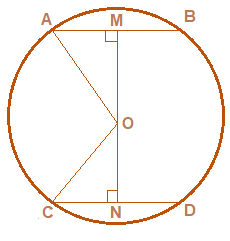From the figure we can say that, AM = MB = 3 cm and CN = ND = 6 cm

Because, perpendicular from centre to a chord bisects the chord.

Let us assume, OA = OC = p

OM = y

ON = 3 – y

Consider the ΔCNO,

By using Pythagoras theorem, OC2 = ON2 + CN2

p2 = (3 – y)2 + (6)2 … [equation (i)]

Now consider the ΔOMA

By using Pythagoras theorem, OA2 = OM2+ AM2

p2 = y2 + 32 … [equation (ii)]

Combining equation (i) and equation (ii), we get,

(3 – y)2 + 62 = y2 + 32

9 + y2 – 6y + 36 = y2 + 9

By transposing, we get,

9 – 9 + y2 – y2 – 6y + 36 = 0

-6y + 36 = 0

y = 36/6

y = 6

Now substitute the value of y in equation (ii) to find out the value p,

p2 = y2 + 32

p2 = 62 + 32

p2 = 36 + 9

p2 = 45

p = √45

p = 3√5

Therefore, the radius of the circle is 3√5 cm.

8. Two chords of lengths 10cm and 24cm are drawn parallel to each other in a circle. If they are on the same side of the centre and the distance between them is 17cm, find the radius of the circle.

Solution:-From the figure we can say that, AM = MB = 5 cm and CN = ND = 12 cm

Because, perpendicular from centre to a chord bisects the chord.

Let us assume, OA = OC = p

OM = y

ON = 17 – y

Consider the ΔCNO,

By using Pythagoras theorem, OC2 = ON2 + CN2

p2 = (17 – y)2 + (12)2 … [equation (i)]

Now consider the ΔOMA

By using Pythagoras theorem, OA2 = OM2+ AM2

p2 = y2 + 52 … [equation (ii)]

Combining equation (i) and equation (ii), we get,

(17 – y)2 + 122 = y2 + 52

289 + y2 – 34y + 144 = y2 + 25

By transposing, we get,

289 + 144 – 25 + y2 – y2 – 34y = 0

408 – 34y = 0

y = 408/34

y = 12

Now substitute the value of y in equation (ii) to find out the value p,

p2 = y2 + 52

p2 = 122 + 52

p2 = 144 + 25

p2 = 169

p = √169

p = 13

Therefore, the radius of circle is 13.

9. In fig, AB a chord of the circle is of length 18 cm. It is perpendicularly bisected at M by PQ. If MQ = 3 cm, find the length of PQ.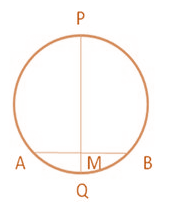Solution:-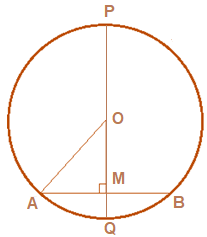From the question it is given that,

Length of chord AB = 18 cm

MQ = 3 cm

From the figure we can say that, AM = MB = 5 cm

Because, perpendicular from centre to a chord bisects the chord.

Let us assume, OA = OQ = p

OM = (r – 3)

Consider the ΔOMA,

By using Pythagoras theorem, OA2 = OM2 + MA2

r2 = (r – 3)2 + 92

r2 = r2 + 9 – 6r + 81

r2 – r2 + 6r = 90

6r = 90

r = 15 cm

Then, PQ = 2r

= 2(15)

= 30 cm

10. AB and CD are two equal chords of a circle intersecting at P as shown in fig. P is joined to O, the centre of the circle. Prove that OP bisects ∠CPB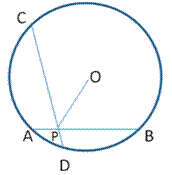Solution:-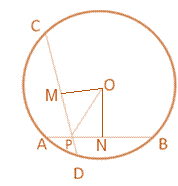Construction: Draw perpendiculars OM and ON to AB and CD, respectively.

Now, consider the ΔOMP and ΔONP,

OP = OP … [common side for both triangles]

OM = ON … [distance of equal chords from the centre are equal]

∠PMO = ∠PNO … [both angles are equal to 90o]

Therefore, ΔOMP ≅ΔONP

So, ∠MPO = ∠NPO

Hence it is proved that, OP bisects ∠CPB.

11. In fig, the centre is O. PQ, and RS are two equal chords of the circle which, when produced, meet at T outside the circle. Prove that (a) TP = TR, (B) TQ = TS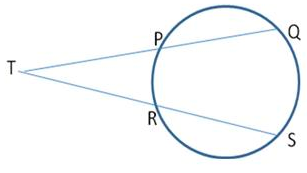Solution:-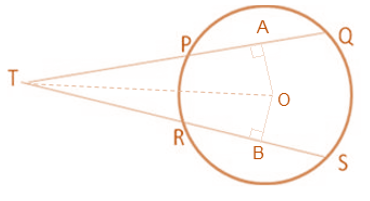From the question it is given that PQ = RS

We have to prove that, TP = TR and TQ = TS

Then, Draw OA ⊥ PQ and OB ⊥ RS,

Since equal chords are equidistant from the circle therefore,

PQ = RS ⇒ OA = OB … [equation (i)]

So, PA = AQ = ½ PQ and RB = BS = ½ RS

Given PQ = RS, we get

PA = RB … [equation (ii)]

And, AQ = BS … [equation (iii)]

Now, consider the ΔTAO and ΔTBO,

TO = TO [common side for both triangles]

AO = BO [from equation (i)]

∠TAO = ∠TBO [both angles are equal to 90o]

Therefore, ΔTAO ≅ ΔTBO [By RHS]

So TA = TB [By CPCT] … [equation (iv)]

By subtracting equation (ii) from equation (iv), we get

TA – PA = TB – RB

⇒ TP = TR

Now adding equation (iii) and equation (iv), we get

TA + TQ = TB + BS

Therefore, TQ = TS

Hence proved

12. PQ and QR are two equal chords of a circle. A diameter of the circle is drawn through Q. Prove that the diameter bisect ∠PQR.

Solution:-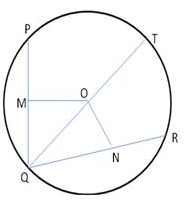let us assume that QT be the diameter of ∠PQR

Then, from question, it is given that PQ = QR

So, OM = ON

Now, consider the ΔOMQ and ΔONQ,

∠OMQ = ∠ONQ …[both angles are equal to 90o]

OM = ON … [from question it is given that equal chords]

OQ = OQ … [common side for both triangles]

Therefore, ΔOMQ ≅ ΔONQ [By RHS]

So, ∠OQM = ∠OQN [CPCT]

Hence QT i.e. diameter of the circle bisects ∠PQR

13. M and N are the midpoints of chords AB and CD. The line MN passes through the centre O. Prove that AB ∥ CD.

Solution:-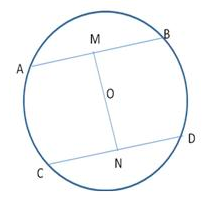From the figure,

AM = MB

CN = ND

Therefore, OM ⊥ AB

Then, ON ⊥ CD

Because, a line bisecting the chord and passing through the centre of the circle is perpendicular to the chord.

So, ∠OMA = ∠OND …[both angles are equal to 90o]

Therefore, AB ∥CD

14. Prove that the line segment joining the midpoints of two parallel chords of a circle passes through its centre.

Solution:-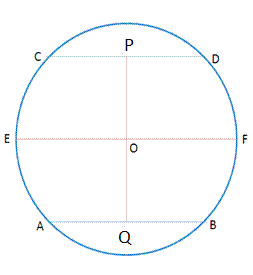Let us assume AB and CD be two parallel chords having Q and P as their mid-points, respectively.

Let O be the centre of the circle.

Join OP and OQ and draw EF || AB | | CD. Since, P is the mid-point of CD.

OP ⊥ CD

∠CPO = ∠DPO = 90o

But OF || CD

∴ ∠POF = ∠CPO … [alternate interior angle]

∠POF = 90o

Similarly, ∠FOQ = 90o

Now, ∠POF + ∠FOQ = 90o + 90o = 180o

Therefore, POQ is a straight line.

Hence proved

15. Two congruent circles have their centres at O and P. M is the midpoint of the line segment OP. A straight line is drawn through M cutting the two circles at the points A, B, C and D. Prove that the chords AB and CD are equal.

Solution:-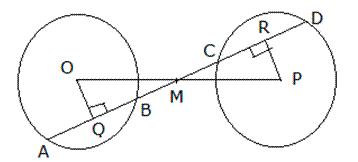From the question, it is given that two congruent circles have their centres at O and P. M is the midpoint of the line segment OP.

We have to prove that chord AB and CD are equal.

Then, draw OQ ⊥ AB and PR ⊥ CD.

Now consider the ΔOQM and ΔPRM

OM = MP … [because M is the midpoint]

∠OQM = ∠PRC …[both angles are equal to 90o]

∠OMQ = ∠PMR …[vertically opposite angles are equal]

Therefore, ΔOQM ≅ ΔPRM [By AAS]

OQ = PR [By CPCT]

The perpendicular distances of two chords in two congruent circles are equal,

Therefore, chords are also equal

So, AB = CD

Hence proved